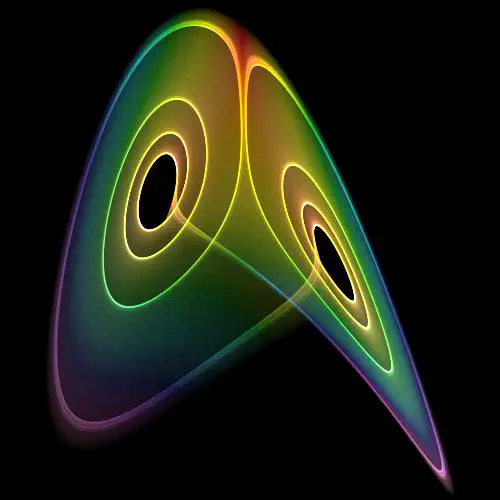# Differential Equations

Umeå University course 5MA121.Image credit: from Wikipedia

This is the basic course 5MA121 in differential equations aimed at second year students. The course covers the formulating and solving first and second order ordinary differential equations. Additional topics include nonlinear dynamics, the Laplace transform, Fourier series, and an introduction to solving partial differential equations. Emphasis is placed on teaching students different ways to model physical and biological systems with differential equations. Students are evaluated by exams and a computer lab that requires the students to use MATLAB to numerically solve differential equations.

The primary text is Elementary Differential Equations and Boundary Value Problems 2017 edition by William Boyce (ISBN 9781119382874). Additional material is drawn from Nonlinear Dynamics and Chaos by Steven Strogatz and Understanding Nonlinear Dynamics by Daniel Kaplan and Leon Glass.##### Eric Libby
###### Associate professor

My research addresses how simple organisms evolve to be complex.